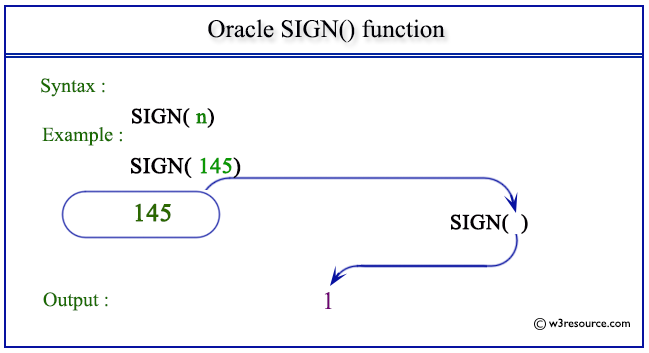# Oracle SIGN() function

## Description

The SIGN() returns the sign of an argument.

This function is used to return the sign of a given number. This function takes as an argument any numeric data type and any nonnumeric data can also comes in the argument but that can be implicitly converted to number and returns number.

The function returns:

• 1 when the value of the argument is positive
• -1 when the value of the argument is negative
• 0 when the value of the argument is 0

For binary floating-point numbers (BINARY_FLOAT and BINARY_DOUBLE), this function returns the sign bit of the number. The sign bit is:

• -1 if n<0
• +1 if n>=0 or n=NaN

Syntax:

`SIGN(n)`

Parameters:

Name Description
n A number whose sign is to be retrieved.

Pictorial Presentation of SIGN() functionExample:

``````SELECT SIGN(-145), SIGN(0), SIGN(145) FROM dual;
```
```

Here is the result.

```SIGN(-145)    SIGN(0)  SIGN(145)
---------- ---------- ----------
-1          0          1
```

The above statement will return the sign of given numbers -145, 0 and 145.

Previous: ROUND
Next: SIN

﻿